MORE IN Elements of Civil Engg. & Engg. Mechanics
VTU First Year Engineering (P Cycle) (Semester 2)
Elements of Civil Engg. & Engg. Mechanics
June 2012
Total marks: --
Total time: --
INSTRUCTIONS
(1) Assume appropriate data and state your reasons
(2) Marks are given to the right of every question
(3) Draw neat diagrams wherever necessary

1 (a) (i) A Bascule bridge is a
(A) Floating bridge
(B) Arch bridge
(C) Suspension bridge
(D) Movable bridge
1 M
1 (a) (ii) Geotechnical engineering involves the study of
(A) Water
(B) Soil
(C) Air
(D) All of these
1 M
1 (a) (iii) Pick ip a structure in which an inspection gallery is formed
(A) Dam
(B) Bridge
(C) Harbour
(D) Ariport
1 M
1 (a) (iv) The part of civil engineering which deals with waste water and solid waste is called
(A) Transporting Engineering
(B) Structural Engineering
(C) Sanitary Engineering
(D) Surveying
1 M
1 (b) Explain the role of civil engineer in the infra-structural development of a nation.
6 M
1 (c) Explain different types of roads.
6 M
1 (d) Give the difference between Earthen dam and gravity dam.
4 M

2 (a) (i) The moment of a force about a moment centre is a measure of its
(A) Translatory effect
(B) Rotational effect
(C) Both A and B
(D) None of these
1 M
2 (a) (ii) Effect of force on a body depends on
(A) Magnitude
(B) Direction
(C) Position
(D) All of these
1 M
2 (a) (iii) Couple means two forces acting parallel and
(A) Equal in magnitude and in same direction
(B) Not equal in magnitude but in same direction
(C) Equal in magnitude but opposite in direction
(D) None of these
1 M
2 (a) (iv) The magnitude of the moment is ______ when a force is applied perpendicular to a lever
(A) Maximum
(B) Minimum
(C) Zero
(D) Negative
1 M
2 (b) State and explain priciple of transmissibility of a force.
4 M
2 (c) Explain equivalent force-couple system.
4 M
2 (d) Determine angle ? (0 ? ? ? 180°) for the force F=200N shown in fig.Q2(d), so that it produces
(A) Maximum moment about 'A' and
Determine maximum and minimum moments.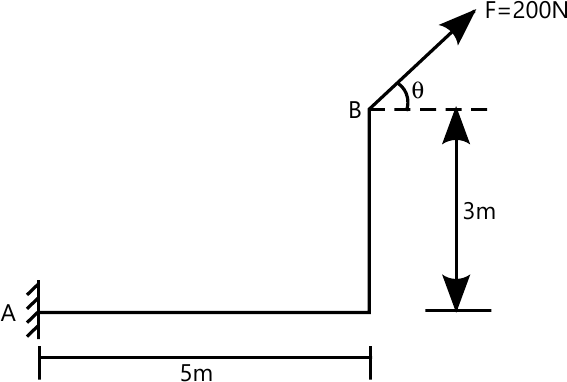8 M

3 (a) (i) The process of finding the resultant of a system of forces is called
(A) Resultant
(B) Resolution
(C) Composition
(D) None of these
1 M
3 (a) (ii) If two forces P and Q (P>Q) act on the same straight line but in opposite direction their resultant is
(A) P+Q
(B) P/Q
(C) Q-P
(D) P-Q
1 M
3 (a) (iii) Component of a force at a right angles to its line of action is
(A) Zero
(B) Positive
(C) Negative
(D) None of these
1 M
3 (a) (iv) In a coplanar concurrent force system if ?H=0, then the resultant is
(A) Horizontal
(B) Vertical
(C) Moment
(D) None of these
1 M
3 (b) The 26kN force is the resultant of two forces, one of which is shown in fig.Q3(b). Determine the other force.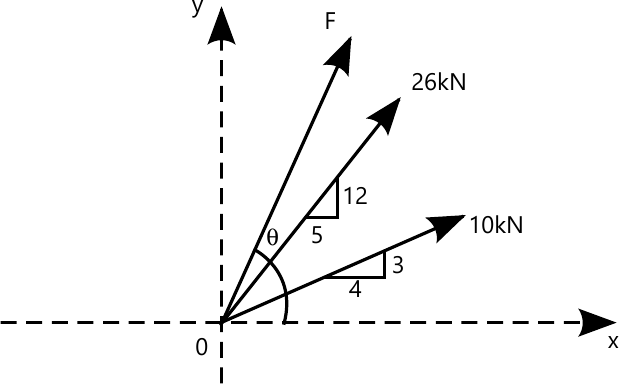8 M
3 (c) A rigid plate is subjected to the forces as shown in fig.Q3(c),compute resultant of forces and position of resultant force with respect to centroid point '0' of the plate.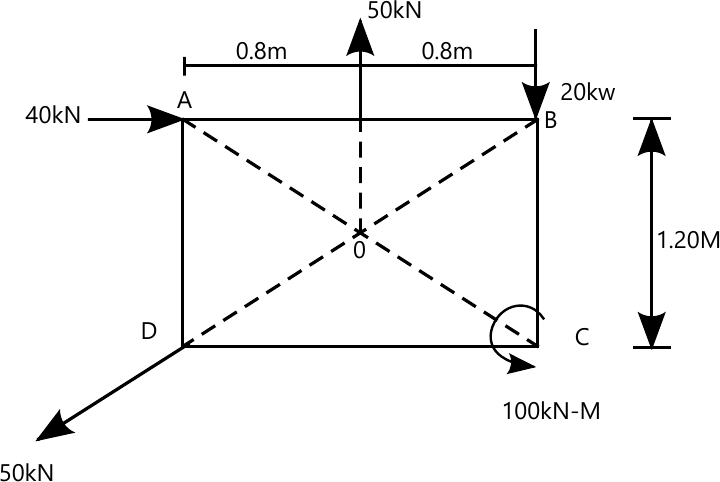8 M

4 (a) (i) Centroid of semicircle of radius 'R' about its centroidal axis parallel to diametric axis is
$(A)\ \dfrac {3R}{4\pi}\\(B)\ \dfrac {3R}{8\pi}\\(C)\ \dfrac {4R}{\pi}\\(D)\ \dfrac {4R}{3\pi}$
1 M
4 (a) (ii) An axis over which one half of plane figure is just mirror image of the other half is
(A) Axis of symmetry
(B) Unsymmetrical axis
(C) Bottom most axis
(D) None of these
1 M
4 (a) (iii) Moment of total area about its centroidal axis is
(A) Twice the area
(B) Three times the area
(C) Zero
(D) None of these
1 M
4 (a) (iv) The centroid of a triangular lamina of height 'h' is situated at a distance ______ from its apex.
$(A)\ \dfrac {h}{3}\\(B)\ \dfrac {2h}{3}\\(C)\ \dfrac {h}{2}\\(D)\ \dfrac {h}{4}$
1 M
4 (b) Locate the centroid of the shaded area shown in fig. Q4(b), with respect to point '0'.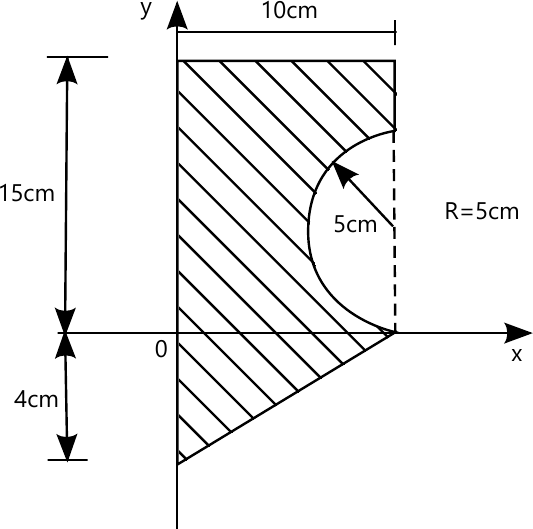8 M
4 (c) The centroid of the rectangular area requires to be shifted from point '0' to 01 (2 cms). This is accomplished by removing hatch portion which is 12cm deep and symmetrical about X X-axis. Determine area of hatched portion shown in fig.Q4(c).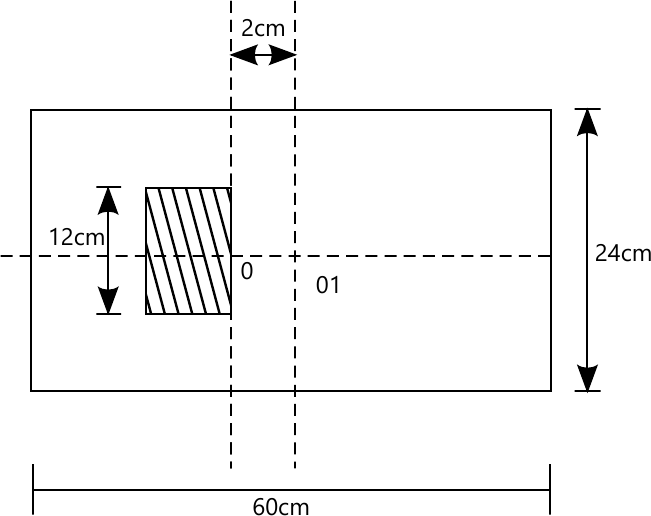8 M

5 (a) (i) The force which is equal and opposite to resultant is
(A) Resultant force
(B) Moment
(C) Equilibrant
(D) None of these
1 M
5 (a) (ii) A particle acted upon by the two forces of equal magnitude is in equilibrium. The angle between the forces is
(A) 0°
(B) 90°
(C) 180°
(D) 45°
1 M
5 (a) (iii) The necessary condition of equilibrium of a coplanar concurrent force system is algebraic sum of ______ must be zero.
(A) Horizontal and Vetical forces
(B) Moment of forces
(C) Horizontal Vertical and moment of forces
(D) None of these
1 M
5 (a) (iv) Lami's equation can be applied when number of unknown forces are ______
(A) Two
(B) Five
(C) Four
(D) Three
1 M
5 (b) Determine the angle ? for the system of strings ABCD in equilibrium as shown in fig.Q5(b).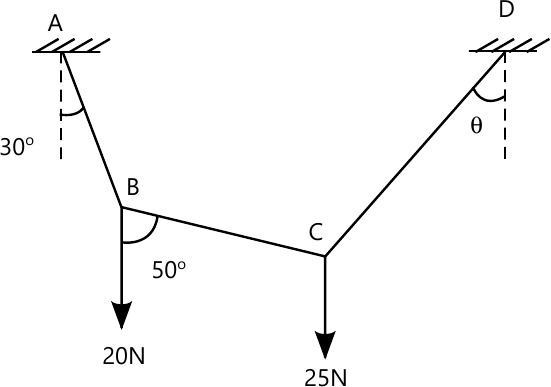8 M
5 (c) A cylinder of weight 600N rests on smooth surfaces as shown in fig.Q5(c). Determine the reactions at contact points. The contact surfaces are prependicular to each other.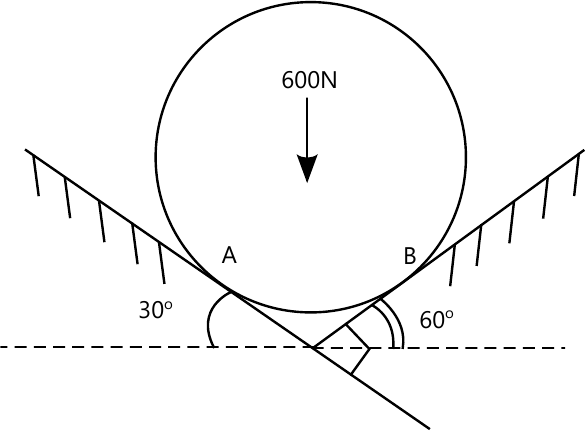8 M

6 (a) (i) A cantilever beam is one in which _______
(A) Both ends are fixed
(B) Both ends are hinged
(C) One end is fixed and other end is simply supported
(D) One end is fixed and other end is free
1 M
6 (a) (ii) A truss is perfect when
(A) m=2j-3
(B) 2j=m+3
(C) m=3j-2
(D) 2j=m-3
1 M
6 (a) (iii) The minimum number of members to form a perfect truss is
(A) 1
(B) 2
(C) 3
(D) 4
1 M
6 (a) (iv) The number of reaction components at an hinged end of a beam
(A) Zero
(B) 2
(C) 3
(D) 1
1 M
6 (b) A pin joined truss is loaded and supported as shown in fig.Q6(b). Determine forces in members, BC, GF and CG and nature of forces. Use method of section.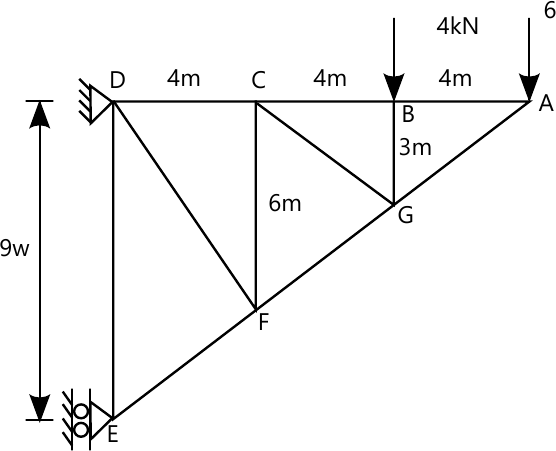8 M
6 (c) Find the reactions for the beam supported and loaded as shown in fig.Q6(c).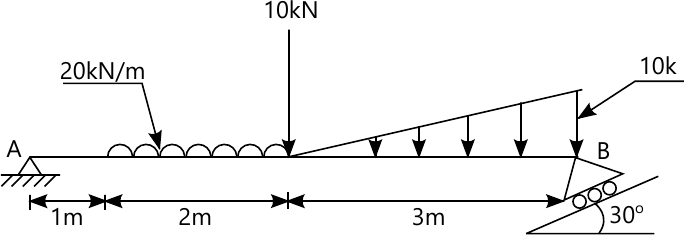8 M

7 (a) (i) Compared to static friction, kinetic friction is
(A) Greater
(B) Smaller
(C) Very large
(D) Zero
1 M
7 (a) (ii) Frictional force acts ______ to the surface in contact
(A) Tangential
(B) Normal
(C) Inclined
(D) None of these
1 M
7 (a) (iii) The force of friction depends upon
(A) Area of contact
(B) Roughness of surfaces
(C) Both area of contact and roughness of surfaces
(D) None of these
1 M
7 (a) (iv) At the point of impending motion, the static frictional forces is
(A) Zero
(B) Maximum
(C) Minimum
(D) Infinite
1 M
7 (b) State laws of static friction.
4 M
7 (c) Briefly explain
(A) Angle of repose
(B) Cone of friction.
4 M
7 (d) A ladder of 7m long weighing 300N is resting against a wall at an angle of 60° to the horizontal ground. A man weighing 700N climbs the ladder, at what position does he induce slipping. Take ?=0.25 for all contact surfaces.
8 M

8 (a) (i) Moment of inertia of a square of side 'b' about an axis through its centroid is
$(A)\ \dfrac {b^4}{12}\\(B)\ \dfrac {b^4}{8}\\(C)\ \dfrac {b^4}{36}\\(D)\ \dfrac {b^3}{12}\\$
1 M
8 (a) (ii) Moment of inertia of a triangle of base 'b' and height 'h' about its base is
$(A)\ \dfrac {bh^3}{36}\\(B)\ \dfrac {bn^4}{36}\\(C)\ \dfrac {hb^3}{12}\\(D)\ \dfrac {Bh^3}{12}\\$
1 M
8 (a) (iii) The unit of radius of gyration is
(A) mm
(B) mm2
(C) KN-m
(D) mm4
1 M
8 (a) (iv) Which of the following equation relating to radii of gyration is correct?
$(A)\ K_{zz}=K_{xx}+K_{yy}\\(B)\ K_{xx}=K_{yy}+K_{zz}\\(C)\ K^2_{zz}=K^2_{xx}+K^2_{yy}\\(D)\ None \ of \ these$
1 M
8 (b) State and prove parallel axis theorem :
6 M
8 (c) Determine moment of inertia and radius of gyration of the area shown in fig.Q8 (c), about base AB and centroidal axis parallel to AB.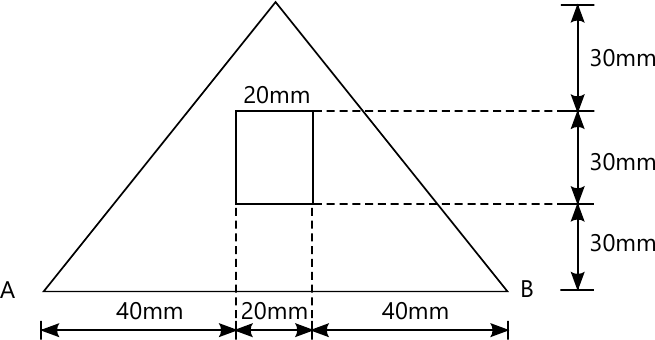10 M

More question papers from Elements of Civil Engg. & Engg. Mechanics